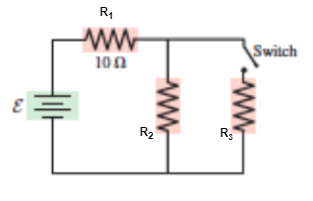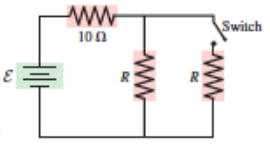# Problem: In the circuit at right, the battery has a voltage of 6.0 V, and the two resistors have resistance 20 Ω each.(a) What is the current in the circuit and the potential difference across the 10 Ω resistor when the switch is open?(b) When the switch is closed?

###### FREE Expert Solution

Equivalent resistance for resistors in parallel for 2 resistors:

$\overline{){{\mathbf{R}}}_{{\mathbf{eq}}}{\mathbf{=}}\frac{{\mathbf{R}}_{\mathbf{1}}{\mathbf{R}}_{\mathbf{2}}}{{\mathbf{R}}_{\mathbf{1}}\mathbf{+}{\mathbf{R}}_{\mathbf{2}}}}$

Equivalent resistance for resistors in series:

$\overline{){{\mathbf{R}}}_{{\mathbf{eq}}}{\mathbf{=}}{{\mathbf{R}}}_{{\mathbf{1}}}{\mathbf{+}}{{\mathbf{R}}}_{{\mathbf{2}}}{\mathbf{+}}{\mathbf{.}}{\mathbf{.}}{\mathbf{.}}{\mathbf{+}}{{\mathbf{R}}}_{{\mathbf{n}}}}$

Current:

$\overline{){\mathbf{i}}{\mathbf{=}}\frac{\mathbf{V}}{\mathbf{R}}}$

Relabel the resistors as:83% (369 ratings)###### Problem Details

In the circuit at right, the battery has a voltage of 6.0 V, and the two resistors have resistance 20 Ω each.
(a) What is the current in the circuit and the potential difference across the 10 Ω resistor when the switch is open?
(b) When the switch is closed?